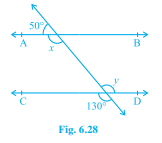"
">

# In Fig. 6.28, find the values of $x$ and $y$ and then show that $\mathrm{AB}=\mathrm{CD}$."

To do:

We have to find the values of $x$ and $Y$ and then show that $AB \parallel CD$.

Solution:

We know that,

The sum of the measures of the angles in linear pairs is always $180^o$.

Since $x$ and $50^o$ are linear pairs of $AB$.

We get,

$x+50^o=180^o$

This implies,

$x=180^o-50^o$

Therefore,

$x=130^o$

We also know that,

Vertically opposite angles are equal.

Therefore, we get $y=130^o$  (since $X$ and $Y$ are vertically opposite angles)

Therefore,

$x=y=130^0$

We know that,

If alternate interior angles are equal, then the two lines are parallel.

Since,

$x$ and $y$ are alternate interior angles and are equal to one another

We get,

$AB \parallel CD$

Therefore,

$AB \parallel CD$

Hence proved.

Updated on: 10-Oct-2022

18 Views